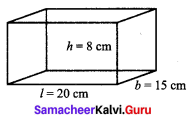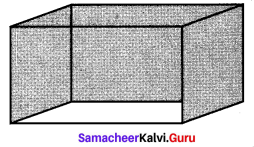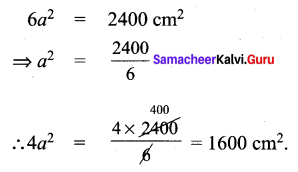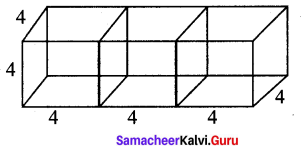## Tamilnadu Samacheer Kalvi 9th Maths Solutions Chapter 7 Mensuration Ex 7.2

9th Maths Exercise 7.2 Samacheer Kalvi Question 1.
Find the total surface area and the lateral surface area of a cuboid whose dimensions are
(i) length = 20 cm,
height = 8 cm
Solution:(i) (a) Total surface Area of cuboid = 2(lb + bh + hl) sq. units
= 2(20 × 15 + 15 × 8 + 8 × 20)
= 2(300 + 120 + 160) = 2 × 580
(b) Lateral surface area of a cuboid = 2h(l + b) sq. units
= 2 × 8 (20 + 15) = 16 × 35 = 560 cm2

9th Maths Mensuration Exercise 7.2 Question 2.
The dimensions of a cuboidal box are 6 m × 400 cm × 1.5 m. Find the cost of painting its entire outer surface at the rate of ₹ 22 per m2
Solution:
l × b × h = 6 m × 400 cm × 1.5 m
l = 6m,
b = 4 m,
h = 1.5 m
∴ Total surface area of the cuboid = Outer surface area
= 2(lb + bh + hl) = 2((6 × 4) + (4 × 1.5) + (1.5 × 6))
= 2(24 + 6 + 9) = 2(39) m2
Cost of painting 1 m2 = ₹ 22
Cost of painting 78 m2 = 78 × 22 = ₹ 1716

10th Maths Exercise 7.2 Samacheer Kalvi Question 3.
The dimensions of a hall is 10 m × 9 m × 8 m. Find the cost of white washing the walls and ceiling at the rate of ₹ 8.50 per m2.
Solution:Dimensions of a hall 10m × 9m × 8m
l = 10 m
b = 9m
h = 8 m
White washing to be done for the area of the surface
= 2 (lh + bh) + lb
= 2 (10 × 8 + 9 × 8) + 10 × 9
= 2 (80 + 72) + 90 = 2 × 152 + 90
= 304 + 90 = 394 m2
Cost of white washing per m2 = ₹ 8.50
Cost of white washing 394 m2 = 394 × 8.50
Total cost = ₹ 3349

9th Maths Exercise 7.2 Question 4.
Find the TSA and LSA of the cube whose side is
(i) 8 m
(ii) 21 cm
(iii) 7.5 cm
Solution:
(i) side of a cube = 8m
TSA of the cube = 6a2 = 6 × 64 = 384 m2
LSA of the cube = 4a2= 4 × 64 = 256 m2
(ii) side a 21 cm
TSA = 6a2 = 6 × 21 × 21 = 2646 cm2.
LSA = 4a2 = 4 × 21 × 21 = 1764 cm2.
(iii) side a = 7.5 cm
TSA = 6a2 = 6 × 7.5 × 7.5 cm2 = 337.5 cm2
LSA = 4a2 = 4 × 7.5 × 7.5 cm2 = 225 cm2

9th Maths 7.2 Question 5.
If the total surface area of a cube is 2400 cm2 then, find its lateral surface area.
Solution:9th Standard Maths Exercise 7.2 In Tamil Question 6.
A cubical container of side 6.5 m is to be painted on the entire outer surface. Find the area to be painted and the total cost of painting it at the rate of ₹ 24 per m2.
Solution:
a = 6.5 m
6a2 = 6 × 6.5 × 6.5 = 253.5 m2
Area to be painted = 253.5 m2
Cost of painting 1 m2 = ₹ 24
∴ Cost of painting 253.5 m2 = 253.5 × 24 = ₹ 6084

9th Maths Exercise 7.2 In Tamil Question 7.
Three identical cubes of side 4 cm are joined end to end. Find the total surface area and lateral surface area of the new resulting cuboid.
Solution:
a = 4 cm
TSA of the cuboid = 2(lb + bh + hl)l = 12 cm
b = 4 cm
h = 4 cm
∴ TSA = 2(12 × 4 + 4 × 4 + 4 × 12)
= 2(48 + 16 + 48) = 2 × 112 = 224 cm2
LSA = 2h(l + b) = 2 × 4(12 + 4) = 8 × 16 = 128 cm2